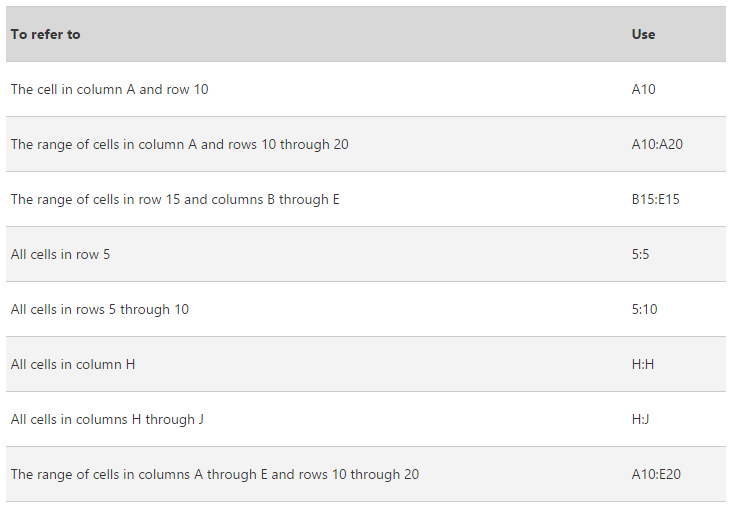## How to Use Cell References in Excel Formulas## We recently looked at Excel formulas from a very introductory standpoint: using Excel for simple calculations with new and existing data.

If you’re ready to start building more advanced formulas, the possibilities are endless. But one prerequisite, whether simple or more complex, is to understand how Excel uses cell references in formulas. The video above gives a brief overview. Read on for more.

A cell reference is just what it sounds like. In Excel formulas, you can reference a particular cell or range of cells and the data in that cell will be used in the calculation. Here’s how to refer to the cell:

By default, Excel uses the A1 reference style, which refers to columns with letters (A through XFD, for a total of 16,384 columns) and refers to rows with numbers (1 through 1,048,576). These letters and numbers are called row and column headings. To refer to a cell, enter the column letter followed by the row number. For example, B2 refers to the cell at the intersection of column B and row 2.

After beginning to type the formula using an equals sign, you can either type the cell label (i.e. =A4 is a reference to the value of cell A3) or use your cursor to select a cell or range of cells. According to Microsoft, “When selecting a range of cells, you can drag the border of the cell selection to move the selection, or drag the corner of the border to expand the selection.”

=A1:A12 is a reference to the range of cells A1 through A12. The colon is considered the “reference operator.”

Take a look at the table below for more types of cell references:## Relative vs. Absolute Cell References

A reference to a cell like =A1 is considered relative, because if the position of the cell that contains the formula is moved, the reference is changed as well. In other words, if your formula referencing =A1 is moved down two rows, it will now reference A3.

You can use absolute references to avoid this if it is unwanted. Adding dollar signs to cell references forces them to become absolute. If you change cell reference =A1 to =\$A\$1, and the formula using it is moved, it will continue to reference cell A1.

As a shortcut, you can switch between reference types by pressing F4.

Cell references can be mixed, so that a reference to a column is absolute while the row is relative, or vice versa. \$A1 contains an absolute column reference and a relative row reference. A\$1 contains an absolute row reference and a relative column reference.